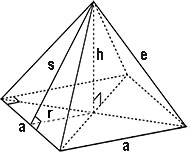# Square Pyramid Calculator

With this Square Pyramid Calculator, you can determine various properties of a square pyramid by inputting only two variables.

A square pyramid is a three-dimensional geometric figure that has a square base and four triangular sides that meet at a point. In simpler terms, it is a pyramid with a square base. The Pyramid of Giza is an example of a square pyramid.

The sides of a square pyramid are made up of four triangles and a square base. The axis runs through the center of the pyramid and is at a right angle to its base.

Pyramid CalculatorYou may set the number of decimal places in the online calculator. By default there are only two decimal places.

Results
Base Surface Area (B):  sq. units
Lateral Surface Area (L):  sq. units
Total Surface Area (A):  sq. units
Volume (V):  cu. units

### The online Square Pyramid Calculator is capable of solving problems that involve the use of the following formulas:

Slant Height (s) = √(h2 + (1/4) a2)

Lateral Surface Area (L) = a √(a2 + 4h2)

Surface Area of Base (B) = a2

Total Surface Area = L + B = a √(a2 + 4h2) + a2

Volume = (1/3)a2h